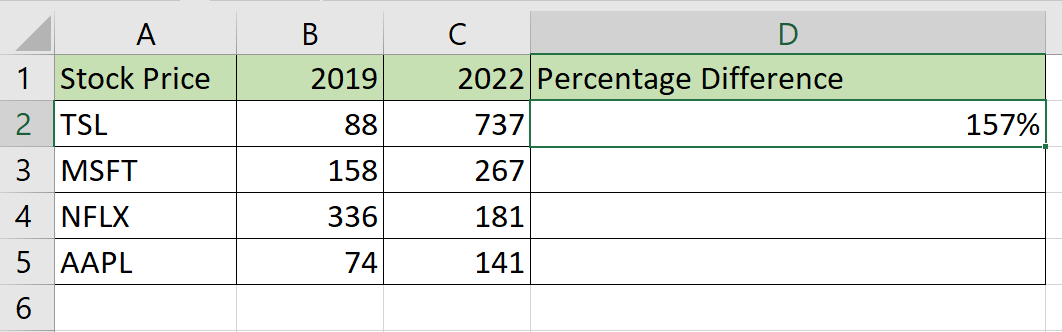# How to Calculate Percentage Difference in Excel

This guide will explain how to calculate the percentage difference in Excel.

Similar to percentage change, the percentage difference is often used when comparing two related values.

We can calculate the percentage difference by finding the absolute difference between two values and dividing it by their average.

For example, the percentage difference between 100 and 175 can be computed using the formula `(175 - 100) / ((175 +100)/2) = 55%`. Percentage difference is always represented as a positive number.

Let’s take a look at a scenario where we can use percentage difference to compare two related values.

Suppose you need to compare test scores between two different classes. Both classes have 30 students, and we will get the average score for each. Class A has an average grade of 85, while Class B has an average grade of 92. How can we calculate the percentage difference in Excel?

We can use the `ABS` and `AVERAGE` functions in a formula to solve for percentage difference. We will need `ABS` since the percentage difference typically ignores negative values. In the next section, we will look into the precise formula to use in Excel.

The percentage difference is best used when comparing values with the same unit. For example, we can use percentage difference to compare objects of different weights or buildings of different heights.

You should not confuse percentage difference with either percentage change or percentage error. The percentage change is simply the increase or decrease of value written as a percentage. Meanwhile, the percentage error is the difference between the estimated and actual values.

Now that we know when to find the percentage difference in Excel, let’s look at a sample spreadsheet that uses it.

## A Real Example of Calculating Percentage Difference in Excel

Let’s take a look at some sample sheets that compare values using percentage differences in Excel.

The example below compares the percentage difference between stock prices in 2019 and 2022. We can use this formula to determine which stocks have grown the most in value. From this example, we can determine that Tesla stock has had the largest difference between its 2019 price and its 2022 price.To get the values in Column D, we just need to use the following formula:

`=ABS(B2-C2)/AVERAGE(B2,C2)`

In this second example, we’ve used percentage difference to compare the performance of two different college classes. From our table, we’ve determined that the classes had a larger difference in the subject of Math than in History.If you’re ready to try using percentage difference to compare values in Excel, follow our guide in the next section!

## How to Calculate Percentage Difference in Excel

This section will guide you through each step needed to start using the percentage difference formula. You’ll learn how we can use the `ABS` and `AVERAGE` function to get the difference and average of the two given values.

Follow these steps to start using percentage difference:

1. First, select the cell that will hold the percentage difference formula. In this example, we’ll start with cell D2.2. Next, we’ll need to compute the absolute difference between our two values. We can use the `ABS` function to convert a possible negative result.3. After computing for the difference, we must divide the result by the average of the two values. In this example, we’ll use the `AVERAGE` function and add both values as arguments.4. Hit the Enter key to evaluate the formula. In our first entry, we’ve determined that the percentage difference between the 2019 and 2022 price is 157%.5. We can use the Fill Handle feature to auto-complete the rest of the column by dragging down the first cell.1. Why do we average both values when computing the percentage difference?
When comparing two values, it is often unclear which value to use as a reference point. For example, if we were to solve for the percentage difference between 20 and 30, we might arrive at a result of either 33% or 50%, depending on which value you place as the denominator. Since we do not have a reference value, solving for the average of the two given values is a compromise.
2. Why do we ignore negative values?
Negative values are ignored because we do not have a reference value for percentage differences. Though the order matters when we subtract values to get the difference, we only need to know the absolute value of this result.

This step-by-step Excel guide should be all you need to start computing the percentage difference between two values. You now know how to compare two related values easily.

The percentage difference is just one example of a metric you can solve for in Excel. With so many other Excel functions available, you can surely find one that suits your use case.

Are you interested in learning more about what Excel can do? Subscribe to our newsletter to learn about the latest Excel guides and tutorials from us.### Get emails from us about Excel.

Our goal this year is to create lots of rich, bite-sized tutorials for Excel users like you. If you liked this one, you'd love what we are working on! Readers receive ✨ early access ✨ to new content.

##### You May Also Like## How to Use SUBTOTAL with COUNTIF in Excel

This guide will discuss how to use SUBTOTAL with COUNTIF in Excel. The rules for using the COUNTIF…## How to Create a Covariance Matrix in Excel

This guide will explain how to create a covariance matrix in Excel. The covariance matrix is a useful…## How To Use AVERAGEIF With Multiple Ranges in Excel

This guide will explain how to use the AVERAGEIF function with multiple ranges in Excel. We will present…## How to Use ANOVA Two Factor Without Replication in Excel

This guide will explain how to create a two-factor ANOVA analysis without replication in Excel. This type of…